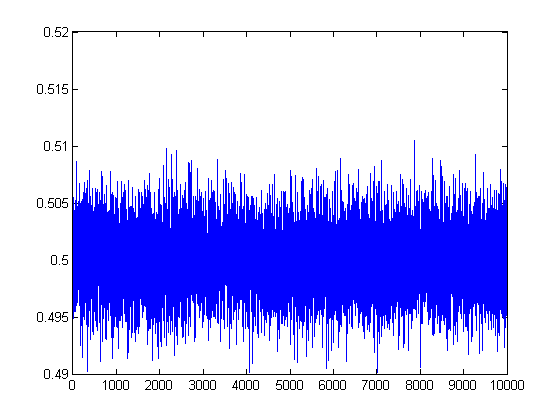# New MAT-File Functionality in R2011b

Today I’d like to introduce guest blogger Sarah Wait Zaranek who works for the MATLAB Marketing team here at The MathWorks. Sarah previously has written about speeding up code from a customer to get acceptable performance. She and I will be writing about the new capabilities for MAT-files in R2011b.

### What's New for MAT-Files?

The new matfile function in R2011b allows you to efficiently load or save to parts of variables to MAT-Files. For you who are running into memory limits, loading part of a variable requires less memory than loading the entire contents of that variable. Previously, there was a way to read in separate variables from a MAT-file but not load parts of a single variable. But now - it is possible to load in parts of your variable. Let’s see this in action!

We have a MAT-file (myBigData.mat) that contains the variable X. You can use the following to to see what variables are available in your MAT-file

whos -file myBigData
  Name          Size                   Bytes  Class     Attributes

X         10000x10000            800000000  double



Wow - X is almost a gigabyte in size. Perfect for trying out this new partial read functionality.

To read in part of a variable, first you create a object that corresponds to a MAT-File, such as

matObj = matfile('myBigData.mat');

By running whos you can see that matObj is a matlab.io.MatFile object:

whos matObj
  Name        Size            Bytes  Class                Attributes

matObj      1x1               112  matlab.io.MatFile



Now you can access variables in the MAT-file as properties of matObj, with dot notation. This is similar to how you access the fields of structures in MATLAB.

loadedData = matObj.X(1:4,1:4);
disp(loadedData)
      0.90579      0.35507      0.89227      0.31907
0.12699        0.997       0.2426      0.98605
0.91338      0.22417       0.1296      0.71818
0.63236      0.65245      0.22507      0.41318


This now loads the data into the workspace. Indices can be a single value, a range of values, or a colon (:). However, note that using the end syntax causes MATLAB to load the entire variable in to memory.

Index this way

[nrows, ncols] = size(matObj, 'X');
loadedData = matObj.X(nrows-10:nrows, ncols-10:ncols);

loadedData2 = matObj.X(end-10:end, end-10:end);

You can now treat your loaded data just as you would any data in MATLAB. Let’s calculate and plot the row average of our data. Every read has a bit of an overhead, so we want to balance the number of reads and the size of the data we bring into MATLAB's memory.

[nrows, ncols] = size(matObj,'X');
dataAvg = zeros(nrows,1);
stepSize = 100;

for ii =1:stepSize:nrows
end

plot(dataAvg)### Example: Using Partial Writing

Again we create an object that corresponds to a MAT-File but focus on writing instead of reading data.

matObj = matfile('myBigData2.mat','Writable',true);

For existing files you will need to set the ‘Writable’ flag to allow you to write to the file. For new files, the default behavior is to allow write permissions.

To partially write to a MAT-file, replace the existing data by the new data just as you normally would for variables in MATLAB.

matObj.X(81:100,81:100) = magic(20);

If you do not index into the variable, the full variable is replaced.

You can also create variables or append to existing data

whos -file myBigData2

matObj.NewVar = rand;
matObj.X(:,10001) = rand(10000,1);

whos -file myBigData2
  Name          Size                   Bytes  Class     Attributes

X         10000x10000            800000000  double

Name            Size                   Bytes  Class     Attributes

NewVar          1x1                        8  double
X           10000x10001            800080000  double



### Format and Indexing Limits

matfile only supports partial loading and saving for MAT-files in Version 7.3 format. If you index into a variable in a Version 7 or earlier MAT-file, MATLAB warns and temporarily loads the entire contents of the variable. To save as version 7.3 format, use the following syntax:

save('mydata.mat','-v7.3');

matfile does not support linear indexing or multilevel indexing, such as indexing into cells of cell arrays or fields of structure arrays. To save structure fields as separate variables to your MAT-file, you can use the following:

S.a = 'try to save';
S.b = 42;
S.c = magic(10);

save('newstruct.mat', '-struct', 'S','-v7.3')
save('newstruct2.mat', '-struct', 'S', 'a', 'c','-v7.3')

whos -file newstruct.mat
whos -file newstruct2.mat
  Name       Size            Bytes  Class     Attributes

a          1x11               22  char
b          1x1                 8  double
c         10x10              800  double

Name       Size            Bytes  Class     Attributes

a          1x11               22  char
c         10x10              800  double



You can learn more about this new functionality, by reading the matfile reference page or by watching this video. There are known performance issues in some cases when using matfile that are currently being look into here at MathWorks. We will update this blog with suggestions on best practices for performance using matfile later.

### Here's How to Create myBigData

Run the code in MakeData, and when it's complete, make a copy of the large MAT-file myBigData.mat to myBigData2. Then you should be able to run this example with your R2011b installation.

type MakeData
% Create a large data file
matObj = matfile('myBigData.mat','Writable',true);

matObj.X(10000,10000) = 0;

for ii = 1:10
rangmin = (ii-1)*1000 + 1;
rangmax = rangmin + 999;
matObj.X(rangmin:rangmax,1:10000) = rand(1000,10000);
end



### Try it Out

Experiment with this new functionality, and let us know what you think about it by leaving a comment here.

Published with MATLAB® 7.13

|